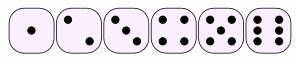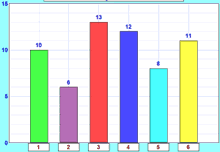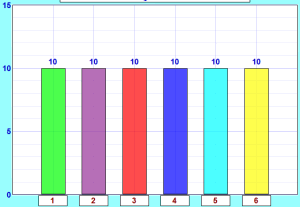# Activity: An Experiment with a Die

 You will need: A single die### Interesting point

Many people think that one of these cubes is called "a dice". But no!

The plural is dice, but the singular is die. (i.e. 1 die, 2 dice.)

The common die has six faces:We usually call the faces 1, 2, 3, 4, 5 and 6.

## High, Low, and Most Likely

Before we start, let's think about what might happen.

Question: If you roll a die:

• 1. What is the least possible score?
• 2. What is the greatest possible score?
• 3. What do you think is the most likely score?

The first two questions are quite easy to answer:

• 1. The least possible score must be 1
• 2. The greatest possible score must be 6
• 3. The most likely score is ... ???

Are they all just as likely? Or will some happen more often?

Let us see which is most likely ...

## The Experiment

Throw a die 60 times,
record the scores in a tally table.

You can record the results in this table using tally marks:

 Score Tally Frequency 1 2 3 4 5 6 Total Frequency = 60

OK, Go!

... ...

... ...

... ...Finished ...?

Now draw a bar graph to illustrate your results.

Or you can use Data Graphs (Bar, Line and Pie) then print it out.You may get something like this:

• Are the bars all the same height?
• If not ... why not?

## 60 Throws

OK, why did I ask you to make 60 throws? Well, 6 throws is not enough for good results. 600 will give good results but is a lot of work. So 60 seems OK, and is also 10 lots of 6.

So we should expect 10 of each number, like this:Those are the theoretical values,
as opposed to the experimental ones you got from your experiment!

How do those theoretical results compare with your experimental results?

This graph and your graph should be similar, but they are not likely to be exactly the same, as your experiment relied on chance, and the number of times you did it was fairly small.

If you did the experiment a very large number of times, you would get results much closer to the theoretical ones.

## Questions

• Which face came up most often? ____
• Which face came up least often? ____
• Do you think you would get the same results if you did this again? Yes / No

An experiment gives results.

When done again it may give different results!

So it is important to know when results are good quality, or just random.

## Probability

On the page Probability you will find a formula:

Probability of an event happening = Number of ways it can happenTotal number of outcomes

### Example: Probability of a 2

We know there are 6 possible outcomes.

And there is only 1 way to get a 2.

So the probability of getting 2 is:

Probability of a 2 = 16

Doing that for each score gets us:

 Score Probability 1 1/6 2 1/6 3 1/6 4 1/6 5 1/6 6 1/6 Total = 1

The sum of all the probabilities is 1

For any experiment:

The sum of the probabilities of all possible outcomes is always equal to 1